Back to blog

# 11 plus maths: everything you need to know

Exam guidesIf your child is applying for a grammar school or a selective independent school for Year 7 entry, it is almost guaranteed that they will sit an 11 plus maths test. At the very least, they will need to answer several questions which test mathematical and problem-solving ability.

Our Education Experts have put together this guide to help you understand what type of questions your child might see in the 11 plus maths exam and how they can use the upcoming months to prepare.

## What are the 11 plus maths topics?

As the exam is taken during Year 6, the 11 plus maths questions children see will be mapped to Level 5 of the UK Key Stage 2 national curriculum (i.e. content taught up until the end of Year 5). The key concepts are number, measurement, geometry and statistics, which can be split down further into 8 subtopics:

### 1. Number: place value

• Read, write, compare, order and round numbers up to 1,000,000

• Count backwards and forwards with positive and negative numbers

• Interpret negative numbers in context

• Read Roman numerals up to 1,000 (M) and recognise years written in Roman numerals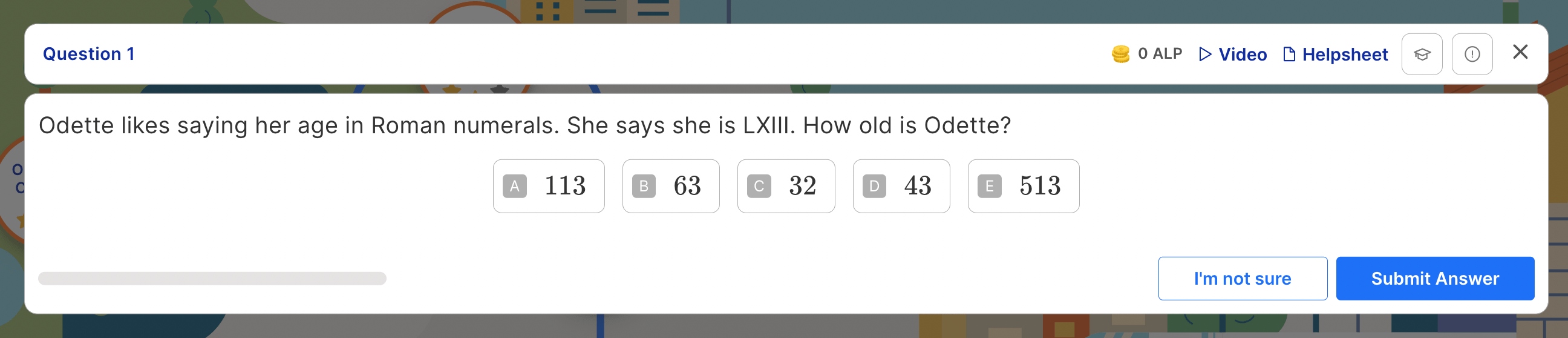### 2. Number: addition and subtraction

• Add and subtract numbers mentally with increasingly large numbers

• Add and subtract whole numbers of more than 4 digits with formal written methods

• Use rounding to check answers and determine levels of accuracy in the context of a problem

• Decide whether to use addition or subtraction when solving multi-step problems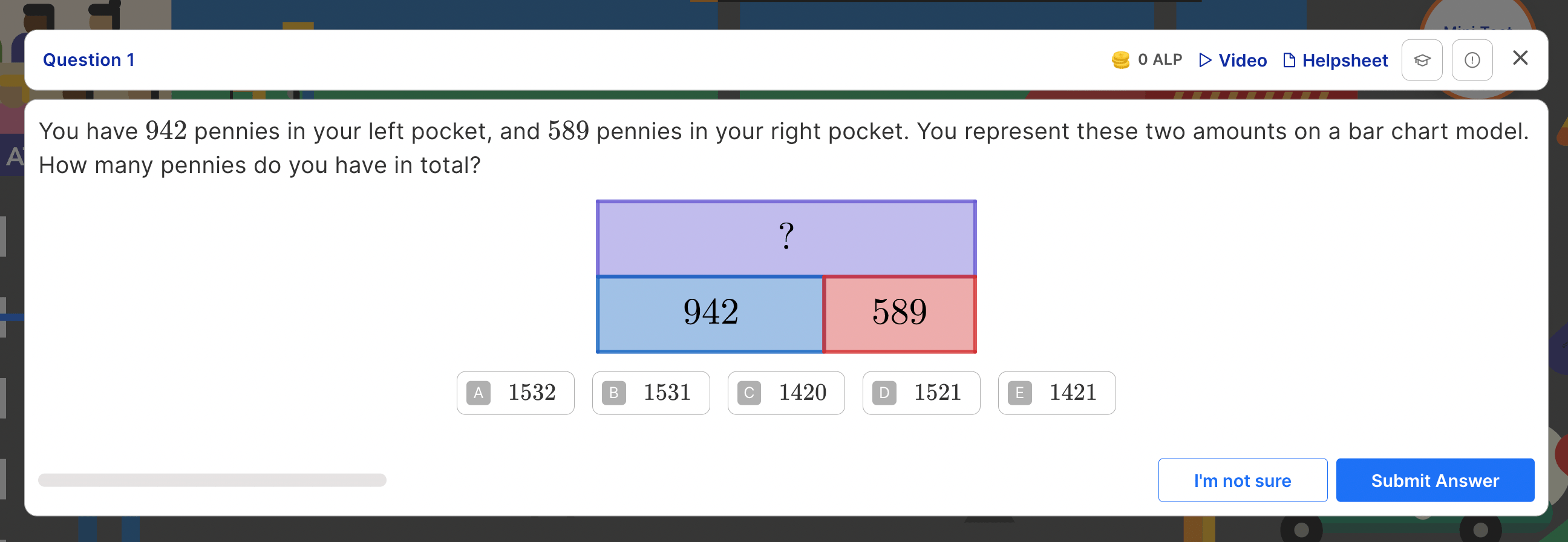### 3. Number: multiplication and division

• Identify multiples, all factor pairs of a number, and common factors of two numbers

• Know and use the vocabulary of prime numbers, prime factors and composite numbers (non-prime numbers)

• Work out whether a number up to 100 is prime and recall prime numbers up to 19

• Use long multiplication when multiplying numbers up to 4 digits by a 2-digit number

• Use short division when dividing numbers up to 4 digits by a 1-digit number

• Identify multiples and factors (including finding common factors of two numbers, and all factor pairs)

• Solve multiplication and division problems using knowledge of factors, multiples, squares and cubes

• Multiply and divide numbers mentally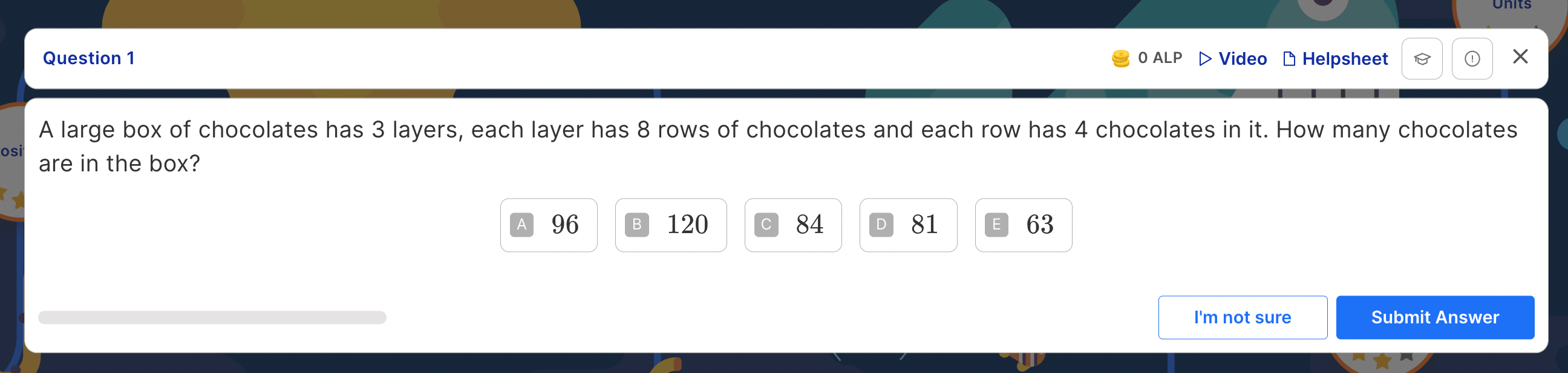### 4. Number: fractions (including decimals)

• Compare and order fractions

• Multiply proper fractions and mixed numbers by whole numbers

• Identify, name and write equivalent fractions

• Add and subtract fractions with the same denominator(s)

• Read and write decimals as fractions

• Compare numbers with up to 3 decimal places, and round decimals with 2 decimal places, to the nearest whole number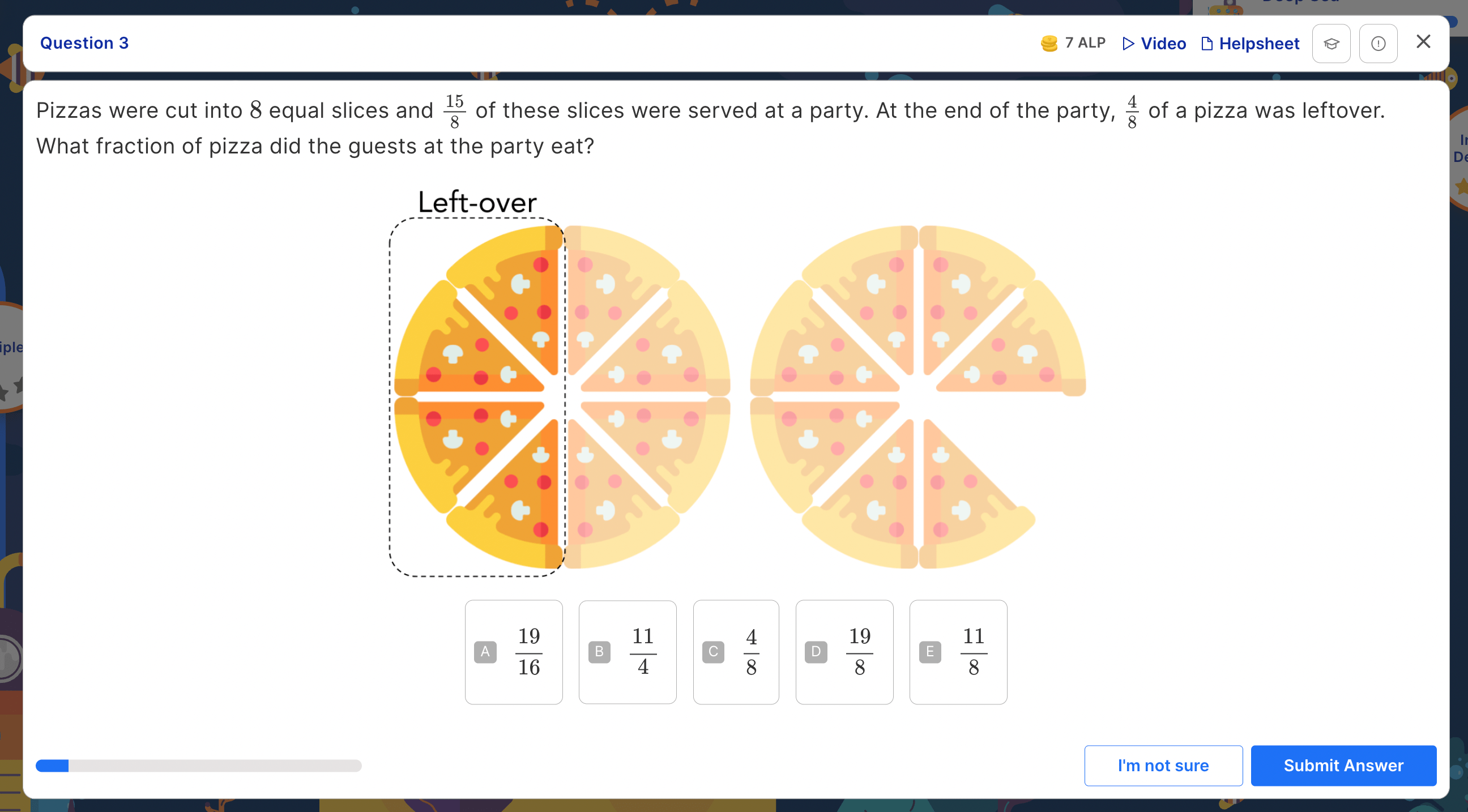### 5. Measurement

• Use appropriate equivalences between metric and imperial units

• Measure and calculate the perimeter of rectilinear shapes in metres and centimetres

• Calculate and compare the areas of rectangles and estimate the area of irregular shapes

• Use standard units, square centimetres and square metres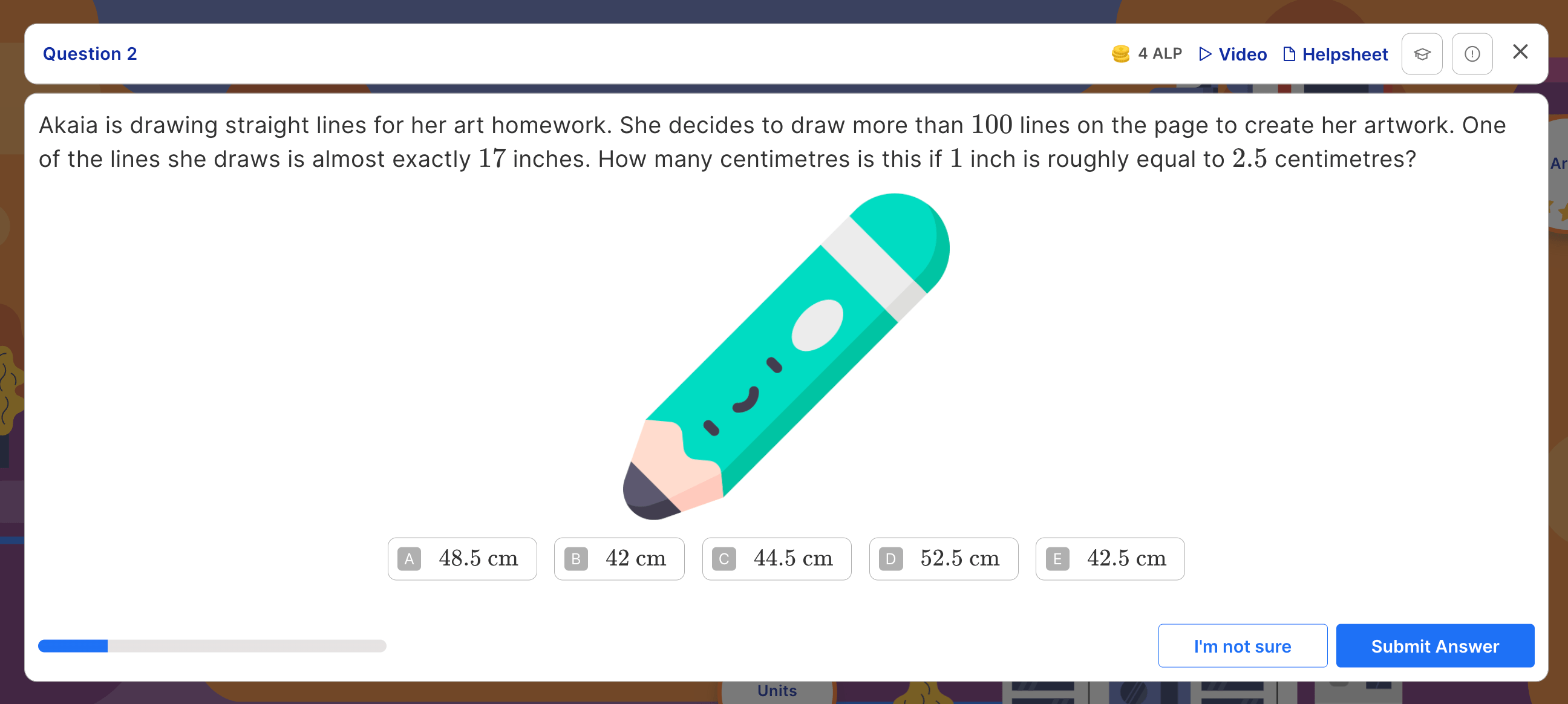### 6. Geometry: properties of shape

• Identify 3D shapes from 2D representations

• Learn how to compare and estimate acute, obtuse and reflex angles

• Distinguish between regular and irregular polygons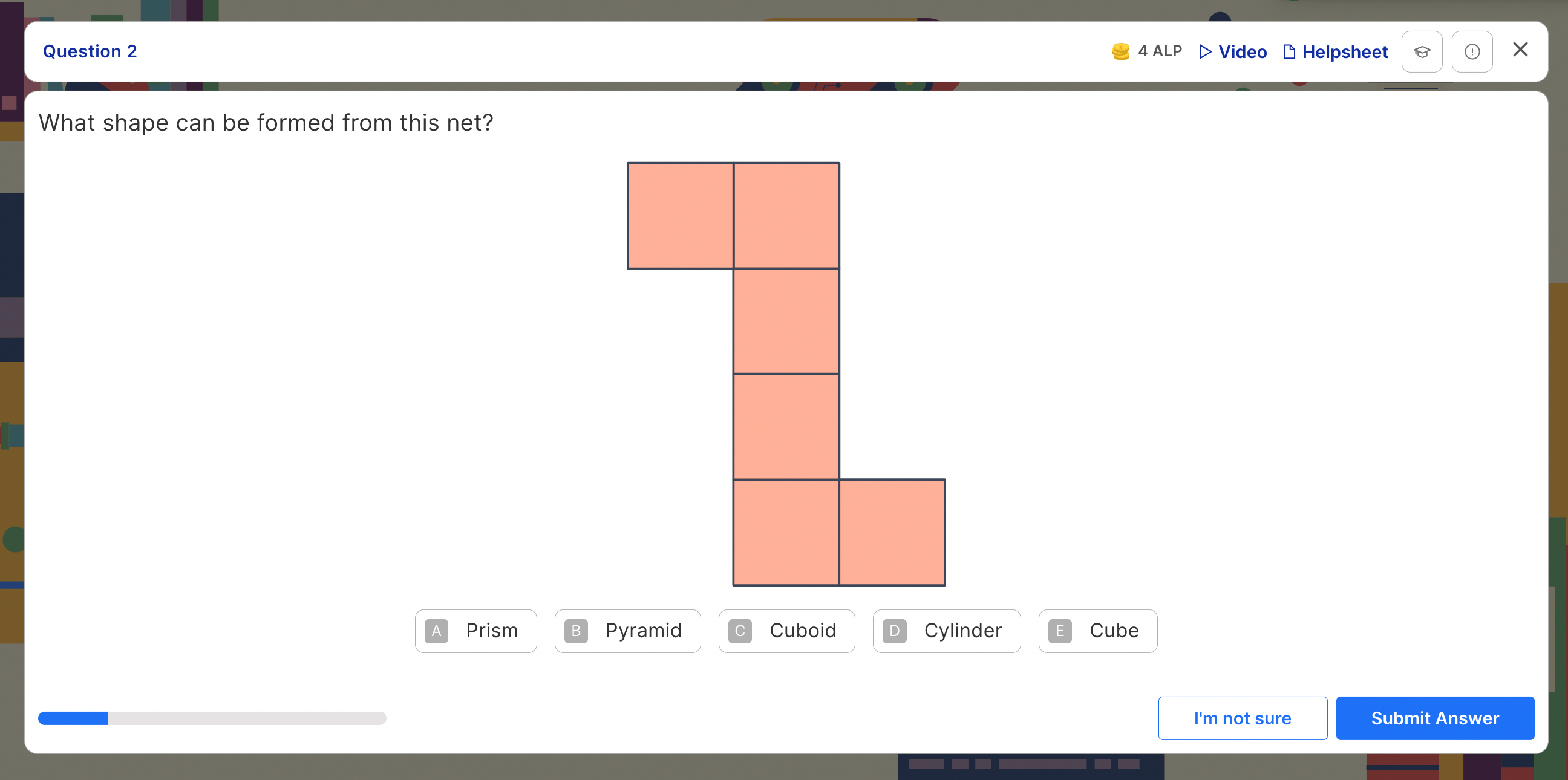### 7. Position and direction

• Reflect shapes and lines

• Identify, represent and describe the position of shapes following a reflection or translation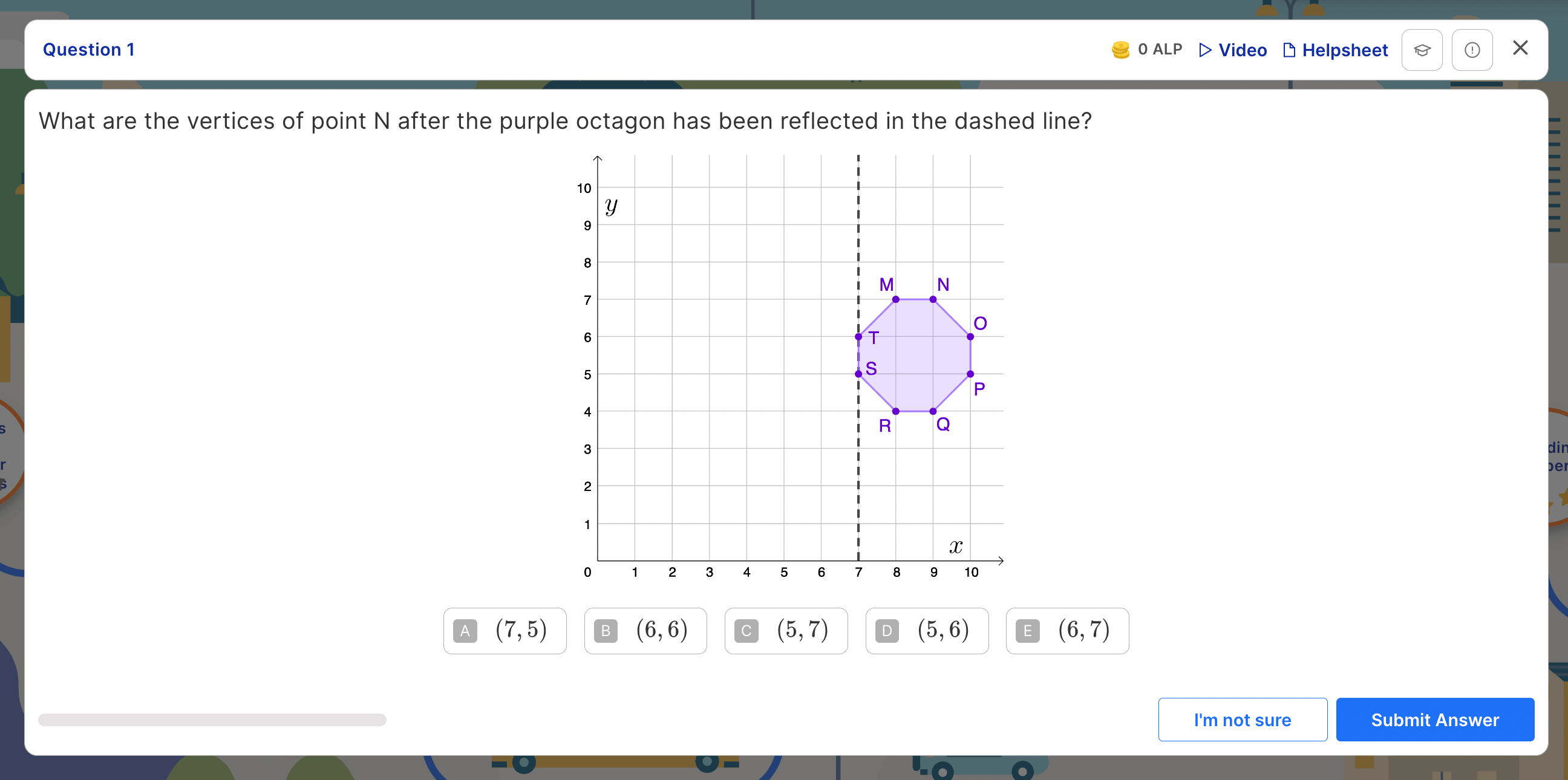### 8. Statistics

• Data-handling – read, complete and interpret data in tables

• Use data presented in a line graph to solve comparison, sum and difference problems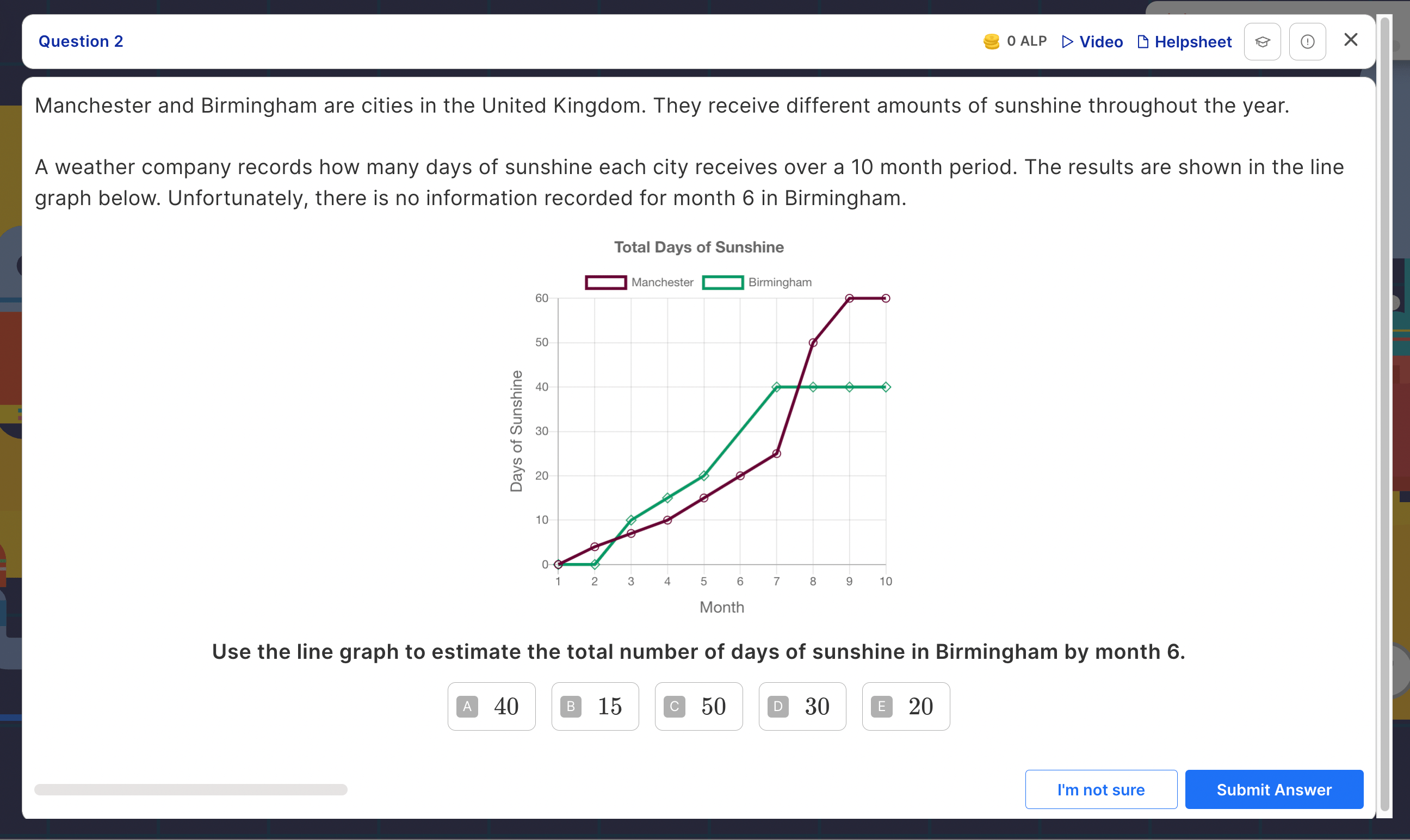## What is the structure of the 11 plus maths exam?

The structure of the 11 plus maths exam will vary depending on which school your child is applying for, and which exam board that school uses.

Most 11 plus maths papers exams will be paper-based, including those administered by CEM (Centre for Evaluation and Monitoring) and GL Assessments (the major exam boards used by grammar schools) and the school itself. However, if your child's target school uses the ISEB (Independent Schools Examination Board), CEM Select or CAT4 test for 11 plus entry, this will be taken online. Regardless of the format, questions may be multiple-choice, standard format (i.e. where children need to manually write or input their answers) or a combination of both.

Regardless of which school your child is applying for, it's highly likely that the maths paper will be non-calculator. Most 11 plus maths exam papers have a time limit of one hour or less.

If your child would like to find out more about the 11 plus maths test from a teacher, register for our free 11 plus past paper walkthrough lesson on Monday 15th August 2022 at 10am. Atom's teachers will explain the content of the maths exam in more depth and give your child tips and advice for how to answer tricky questions. You don't need to be a subscriber to attend!## How is the 11 plus maths test marked?

Each maths question will carry a different number of marks. Typically, many exams will have easier, 1-mark questions at the start of the paper, with the questions becoming increasingly more challenging towards the end of the test. As these later questions will involve multi-step problems, there will likely be more marks available.

Some exam boards may reward pupils with marks for showing their workings, so it’s a good idea to ensure your child gets into the habit of writing down their problem-solving in the area underneath the question.

When the test is marked, the examiner will add all of the marks together to create a raw score. Some exam boards may use standardised marking so that pupils who were born towards the end of the school year are not disadvantaged by age. In the standardisation process, younger pupils (determined by their age in years and months) will be given extra marks as compensation.

Find out more in our guide to what makes a ‘good’ 11 plus score.

## How can my child prepare for the 11 plus maths exam?

As the maths paper covers the content taught by schools in Year 5, it is important that your child has a strong understanding of every key topic before the exam day.

Atom Nucleus is an industry-leading online learning platform with over 90,000 interactive practice questions, covering all core Key Stage 2 topics. Working through each subtopic at a time is a great low-stakes way for your child to consolidate everything they need to know. Every question includes supportive resources with prompts, downloadable helpsheets and video tutorials to ensure that your child fully understands how to solve the problem.

Have a go at some quick 11 plus maths questions now: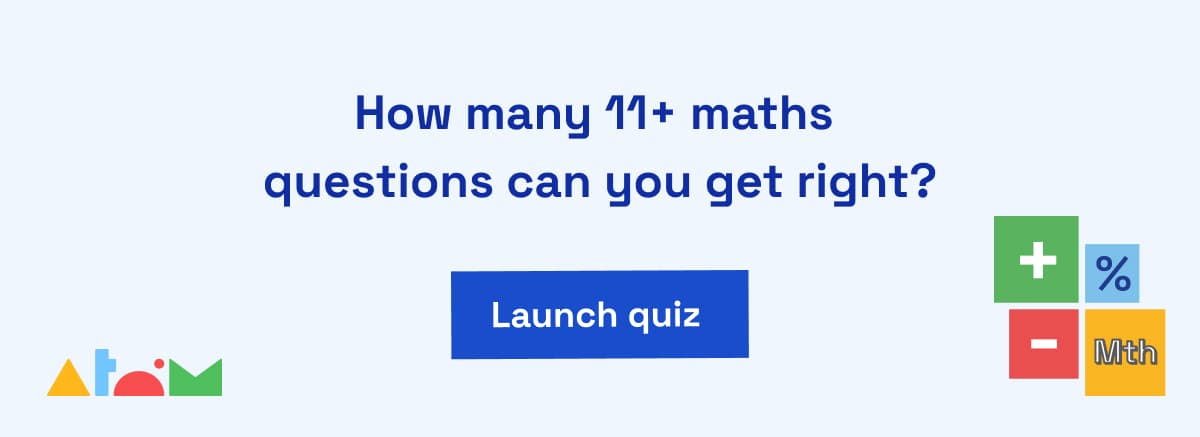## Unlock 11 plus maths practice papers

Once your child is confident with the Year 5 maths curriculum, they will be ready to practise their skills with exam papers.

Atom subscribers can access unlimited online maths tests that replicate real 11 plus maths papers – all on one online platform. Children can take the tests as many times as they like and never see the same questions in the same order twice.

Best of all, there's no need for parents to mark. Atom's mock tests are automatically marked and analysed, with the results clearly displayed on your parent dashboard so you can celebrate your child's strengths and progress and help them identify more challenging topics that need further revision.

Try Atom Nucleus free for 5 days and explore maths learning journeys, unlimited 11 plus maths practice papers and get access to expert advice for admissions to independent and grammar schools.

Start your 5 day free trial

## Looking for more resources?

Discover more ways to help your child with their 11 plus maths preparation with free resources from Atom's Education Experts.Atom

Learning